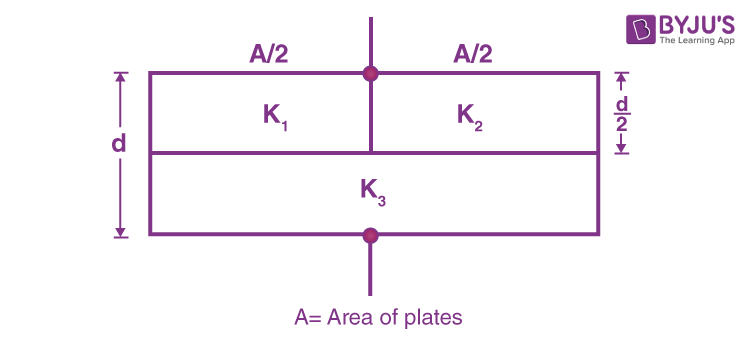# A parallel plate capacitor of area A, plate separation d and capacitance C is filled with three different dielectric materials having dielectric constant K1, K2, K3 as shown. If a single dielectric material is to be used to have the same capacitance C in this capacitor, then its dielectric constant K is given by?

A parallel plate capacitor of area A, plate separation d and capacitance C is filled with three different dielectric materials having dielectric constant K1, K2, K3 as shown. If a single dielectric material is to be used to have the same capacitance C in this capacitor, then its dielectric constant K is given by?1. 1/K=1/K1+1/K2+1/2K3
2. 1/K=1/K1+K2 + 1/2K3
3. 1/K=K1K2/K1+K2 + 2K3
4. K=K1K3/K1+K3 + K2K3/K2+K3

The correct option is iv. K=K1K3/K1+K3 + K2K3/K2+K3(0)(0)